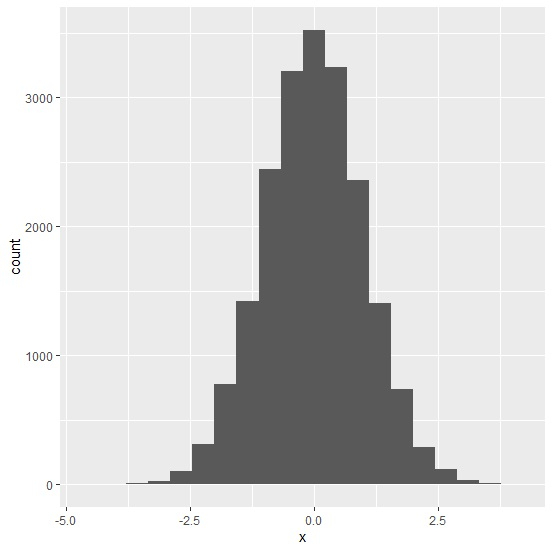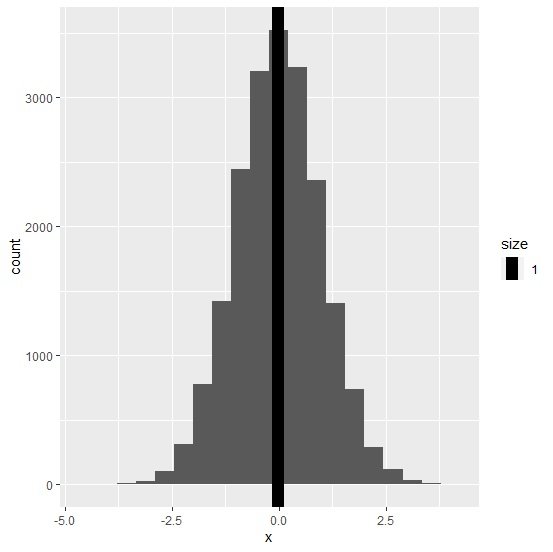# How to display mean in a histogram using ggplot2 in R?

To display mean in a histogram using ggplot2, we can use geom_vline function where we need to define the x-intercept value as the mean of the column for which we want to create the histogram. Also, we can change the size of the line for mean in the histogram by using size argument inside geom_vline function.

Consider the below data frame −

x<-rnorm(20000)
df<-data.frame(x)

## Example

library(ggplot2)
ggplot(df,aes(x))+geom_histogram(bins=20)

## OutputCreating histogram of x with mean displayed on the plot −

## Example

ggplot(df,aes(x))+geom_histogram(bins=20)+geom_vline(aes(xintercept=mean(x),size=1))

## Output